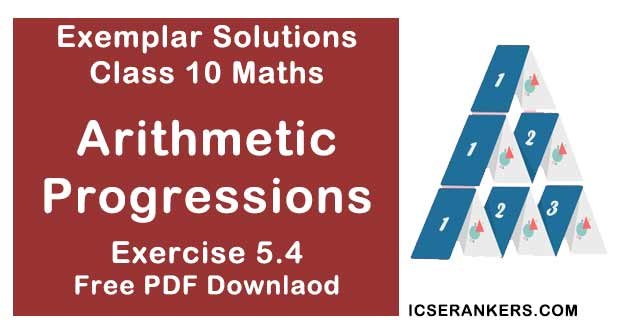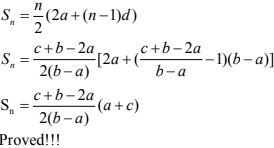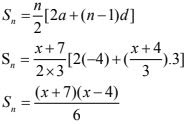# Chapter 5 Arithmetic Progressions NCERT Exemplar Solutions Exercise 5.4 Class 10 MathsChapter Name NCERT Maths Exemplar Solutions for Chapter 5 Arithmetic Progressions Exercise 5.4 Book Name NCERT Exemplar for Class 10 Maths Other Exercises Exercise 5.1Exercise 5.2Exercise 5.3 Related Study NCERT Solutions for Class 10 Maths

### Exercise 5.4 Solutions

1. The sum of the first five terms of an  AP and the sum of the first seven terms of the same AP is 167. If the sum of the first ten terms of this AP is 235, find the sum of its first twenty terms.

Solution

In an A.P. ,
First term = a
Common difference = d
Number of terms of an AP = n
Now, we have,
S5 + S7 = 167
Using the formula,
Sn = n/2 [2a + (n - 1)d]
Putting value,
5/2 [2a + (5 - 1)d] + 7/2 [2a + (7 - 1)d] = 167
⇒ 5(2a + 4d) + 7(2a + 6d) = 334
⇒ 10a + 20d + 14a + 42d = 334
⇒ 24a + 62d = 334
⇒ 12a + 31d = 167
⇒ 12a = 167 - 31d ...(i)
Also,
S10 = 235
⇒ 10/2[2a + (10 - 1)d] = 235
⇒ 5[2a + 9d] = 235
⇒ 2a + 9d = 47
Multiplying 6 in both the sides,
12a + 54d = 282
From equation (i)
167 -31d + 54d = 282
⇒ 23d = 282 - 167
⇒ 23d = 115
⇒ d = 5
Putting the value of d = 5 in equation (i)
12a = 167 – 31(5)
⇒ 12a = 167 – 155
⇒ 12a = 12
⇒ a = 1
Also,
S20 = n/2[2a + (20 - 1)d]
= 20/2 [2(1) + 19(5)]
= 10[2 + 95]
= 970
Hence, the sum of first 20 terms is  970.

2. Find the
(i) Sum of those integers between 1 and 500 which are multiples of 2 as well as of 5.
ii. Sum of those integers from 1 to 500 which are multiples of 2 as well as of 5.
iii. Sum of those integers from 1 to 500 which are multiples of 2 or 5.
[Hint (iii): These numbers will be: multiples of 2 + multiples of 5 – multiples of 2 as well as of 5]

Solution

We know that,
Multiples of 2 as well as of 5 = LCM of (2, 5) = 10
Also, Multiples of 2 as well as of 5 between 1 and 500 = 10, 20, 30..., 490.
We can conclude that 10, 20, 30..., 490 is an AP with common difference,
d = 10
First term,
a = 10
Taking the number of terms in this AP = n
Using nth term formula,
an = a + (n – 1)d
⇒ 490 = 10 + (n – 1)10
⇒ 480 = (n – 1)10
So,
n – 1 = 48
⇒ n = 49
Sum of an AP,
Sn = (n/2)[a + an ]
= (49/2) × [10 + 490]
= (49/2) × 500
= 49 × 250
= 12250
Sum of those integers between 1 and 500 which are multiples of  2 as well as of 5 = 12250

(ii) Sum of those integers from 1 to  500 which are multiples of 2 as well as of 5.

Solution

We have,
Multiples of 2 as well as of 5 = LCM of (2, 5) = 10
Multiples of 2 as well as of 5 from  1 and 500 = 10, 20, 30, ....., 500.
Therefore,
We can conclude that 10, 20, 30, ..... 500 is an AP with common difference,
d = 10
First term, a = 10
Let the number of terms in this AP = n
Using the formula,
an = a +(n - 1)d
⇒ 500 = 10 + (n - 1)10
⇒ 490 = (n - 1)10
⇒ n - 1 = 49
⇒ n = 50
Sum of an AP,
Sn = n/2 [a + an ],
= (50/2)[10 + 500]
= 25 × [10 + 500]
= 25(510)
= 12750
Therefore, sum of those integers from 1 to  500 which are multiples of 2 as well as of  5 = 12750

(iii) Sum of those integers from 1 to 500 which are multiples of 2 or 5.
We know that,
Multiples of 2 or 5 = Multiple of 2 + Multiple of 5 – Multiple of LCM (2, 5)
Multiples of 2 or 5 = Multiple of 2 + Multiple of 5 – Multiple of LCM (10)
Multiples of 2 or 5 from 1 to 500 =
List of multiple of 2 from 1 to 500 + List of multiple of 5 from 1 to 500 – List of multiple of 10 from 1 to 500
= (2, 4, 6... 500) + (5, 10, 15... 500) – (10, 20, 30... 500)
Required sum = sum(2, 4, 6,..., 500) + sum(5, 10, 15,..., 500) – sum(10, 20, 30,., 500)
Consider the first series, 2, 4, 6, ...., 500
First term, a = 2
Common difference, d = 2
Let n be no of terms
an = a + (n – 1)d
⇒ 500 = 2 + (n – 1)2
⇒ 498 = (n – 1)2
⇒ n – 1 = 249
⇒ n = 250
Sum of an AP, Sn = (n/2)[a + an ]
Let the sum of this AP be S1 ,
S1 = S250
= (250/2)[2 + 500]
S1 = 125(502)
⇒ S1 = 62750
Considering the second series,
5, 10, 15, ......, 500
First term,
a = 5,
Common difference,
d = 5
Taking number of terms = n
By the formula
an = a + (n - 1)d
⇒ 500 = 5 + (n - 1)5
⇒ 495 = (n - 1)5
⇒ n - 1 = 99
⇒ n = 100
Sum of an AP,
Sn = (n/2)[a + an ]
Taking the sum of this AP be S2 ,
S2 = S100
= (100/2)[ 5 + 500]
S2 = 50(505)
⇒ S2 = 25250
Considering the third series,
10, 20, 30, ......., 500
First term,
a = 10
Common difference,
d = 10
Taking number of terms = n
an = a + (n - 1)d
⇒ 500 = 10 + (n - 1)10
⇒ 490 = (n - 1)10
⇒ n - 1 = 49
⇒ n = 50
Sum of an AP,
Sn = (n/2)[a + an ]
Taking the sum of this AP be S3 ,
S3 = S50 = (50/2)[2 + 510]
⇒ S3 = 25(510)
⇒ S3 = 12750
Hence, the required Sum,
S = S1 + S2 + S3
⇒ S = 62750 + 25250 - 12750
⇒ S = 75250

3. The eighth term of an AP is half its second term and the eleventh term exceeds one third of its fourth term by 1. Find the 15thterm.

Solution

As we know,
First term of an AP = a
Common difference of AP = d
nth term of an AP,
an = a + (n - 1)d
So,
a8 = (1/2)a2
⇒ 2a8 = a2
⇒ 2(a + 7d) = a + d
⇒ 2a + 14d = a + d
⇒ a = – 13d ...(i)
Also,
a11 = (1/3)a4 + 1
⇒ 3(a + 10d) = a + 3d + 3
⇒ 3a + 30d = a + 3d + 3
⇒ 2a + 27d = 3
Putting a = -13d in the equation,
2(-13d) + 27d = 3
⇒ d = 3
So,
a = -13(3) = -39
Now,
a15 = a + 14d
= -39 + 14(3)
= -39 + 42 = 3
So, 15th term is 3.

4. An AP consists of 37 terms. The sum of the three middle most terms is 225 and the sum of the last three is 429. Find the AP.

Solution

First term of an AP = a
Common difference of AP = d
nth term of an AP,
an = a + (n - 1)d
As,
n = 37(odd),
Middle term will be (n + 1)/2 = 19th term
Hence, the three middle most terms will be, 18th, 19th and 20th terms
Therefore,
a18 + a19 + a20 = 225
Using,
an = a + (n - 1)d
⇒ a + 17d + a + 18d + a + 19d = 225
⇒ 3a + 54d =25
⇒ 3a = 225 - 54d
⇒ a = 75 - 18d
Also, we know that last three terms will be 35th, 36th and 37th terms.
a35 + a36 + a37 = 429
⇒ a + 34d + a + 35d + a + 36d = 429
⇒ 3a + 105d = 429
⇒ a + 35d = 143
Putting a = 75 - 18d from equation (i)
75 – 18d + 35d = 143 (using (i))
⇒ 17d = 68
⇒ d = 4
So,
a = 75 – 18(4)
⇒ a = 3
So, the AP is a, a + d, a + 2d....
Which is 3, 7, 11....

5. Find the sum of the integers between 100 and 200 that are
i. divisible by 9
ii. not divisible by 9
[Hint (ii): These numbers will be: Total numbers – Total numbers divisible by 9]

Solution

(i) The number between 100 and 200 which is divisible by 9 = 108, 117, 126, ...198
Taking the number of terms between 100 and 200 which is divisible by 9 = n
an = a + (n - 1)d
⇒ 198 = 108 + (n - 1)9
⇒ 90 = (n - 1)9
⇒ n - 1 = 10
⇒ n = 11
Sum of an AP = Sn
= (n/2)[a + an ]
Sn = (11/2)[108 + 198]
= (11/2) × 306
= 11 × 153
= 1683

(ii) We know that,
Sum of the integers between 100 and 200 which is not divisible by 9
= (sum of total numbers between 100 and 200) – (sum of total numbers between 100 and 200 which is divisible by 9)
Sum,
S = S1 - S2
In this question,
S1 = Sum of AP 101, 102, 103, -----, 199
S2 = Sum of AP 108, 117, 126, ---- , 198
For AP 101, 102, 103, ----, 199
First term a = 101
Common difference, d = 199
Number of terms = n
Then,
an = a + (n - 1)d
⇒ 199 = 101 + (n – 1)1
⇒ 98 = (n – 1)
⇒ n = 99
Sum of an AP = Sn
= (n/2)[a + an ]
Sum of AP,
S1 = (99/2)[199 + 101]
= (99/2) × 300
= 99(150)
= 14850
For AP 108, 117, 126, ...... 198,
First term,
a = 108
common difference, d = 9
Last term, an = 198
Number of terms = n
Then,
an = a + (n - 1)d
⇒ 198 = 108 + (n - 1)9
⇒ 10 = (n - 1)
⇒ n = 11
Sum of an AP = Sn
= (n/2)[a + an ]
Sum of this AP,
S = (11/2)[108 + 198]
= (11/2) × 306
= 11 × 153
= 1683
Putting the value of S1 and S2 in the equation,
S = S1 - S2
= 14850 - 1683
= 13167

6. The ratio of the 11th term to the 18th term of an AP is 2 :3. Find the ratio of the 5th term to the 21stterm, and also the ratio of the sum of the first five terms to the sum of the first 21 terms.

Solution

Let a and d be the first term and common difference of an AP respectively.
Given,
a11 : a18 = 2 : 3
So, ratio of the sum of the first five terms to the sum of the first 21 terms is S5 : S21
30d : 29d
⇒ (a + 10d)/(a + 17d) = 2/3
⇒ 3a + 30d = 2a + 34d
⇒ a = 4d
a5 = a + 4d
⇒ 4d + 4d
= 8d
a21 = a + 20d
= 4d + 20d
= 24d
So,
ratio = 8d : 24d
= 1 : 3
Now, sum,
S5 = (5/2)[2a + (5 - 1)d]
= (5/2)[2(4d) + 4d]
= 30d
S21 = (21/2)[2a + (21 - 1)d]
= 21/2[2a + 20d]
= 294 d
So, the ratio S5 : S21 is 30d : 294d or 5 : 49

7. Show that the sum of an AP whose first term is a, the second term b and the last term c, is equal to
[(a + c)(b+ c - 2a)]/2(b -a)

Solution

Given, the AP is a, b, c
We have,
First term = a,
Common difference = b -a
Last term (l) = an = 1
= a + (n - 1)d
c = a + (n - 1)(b - a)
(n - 1) = (c - a)/(b - a)
n = (c + b - 2a)/(b - a)
Now,8. Solve the equation
-4 + (-1) + 2 + ..... + x = 437

Solution

We have,
-4 + (-1) + 2 + ... + x = 437 ...(i)
Also,
-4 – 1 + 2 + ...+ x forms an AP with,
First term = -4,
Common difference = – 1 – (-4) = 3
an = 1 = x
nth term of an AP, an = 1
= a + (n - 1)d
x = -4 + (n - 1)3 ...(ii)Also,
Sn = 437
⇒ [(x + 7)(x - 4)]/6 = 437
⇒ x2 + 3x – 2650 = 0
On, solving,
x = 50
or, x = -53
As x cannot be negative, so x = 50

9. Jaspal Singh repays his total loan of Rs 118000 by paying every month starting with the first instalment of Rs 1000. If he increases the instalment by Rs 100 every month, what amount will be paid by him in the 30thinstalment? What amount of loan does he still have to pay after the 30th instalment?

Solution

Total loan taken by Jaspal Singh= ₹ 118000
He repays his total loan by paying every month
His first installment = ₹ 1000
Second installment = 1000 + 100 = ₹ 1100
Third installment = 1100 + 100 = ₹ 1200 and so on
Let its 30th installment be n,
We have 1000, 1100, 1200... which form an AP,
Here,
a = 1000
d = 1100 – 1000 = 100
nth term of an AP,
Tn = a + (n – 1)d
For 30th instalment,
T30 = 1000 + (30 – 1)100
= 100 + 29 × 100
= 1000 + 2900
= 3900
Therefore, ₹ 3900 will be paid by him in the 30th instalment.
He paid total amount upto 30 instalments in ,
1000 + 1100 + 1200 + ........ + 3900
First term (a) = 1000
Last term (l) = 3900
S30 = = 15(1000 + 3900)
= 15 × 4900
= ₹ 73500
Total amount he still have to pay after the 30 installment
= (Amount of loan) – (Sum of 30 installments)
= 118000 – 73500 = ₹ 44500
So, ₹ 44500 still have to pay after the 30th installment.

10. The students of a school decided to beautify the school on the Annual Day by fixing colourful flags on the straight passage of the school. They have 27 flagsto be fixed at intervals of every 2 m. The flags are stored at the position of the middle most flag. Ruchi was given the responsibility of placing the flags. Ruchikept her books where the flags were stored. She could carry only one flag at atime. How much distance did she cover in completing this job and returning back to collect her books? What is the maximum distance she travelled carrying flag?

Solution

The number of flags = 27
Distance between each flag = 2 m.
The flags are stored at the position of the middle most flag which is 14th flag and Ruchi was given the responsibility of placing the flags.
Ruchi kept her books, where the flags were stored which is 14th flag and she coluld carry only one flag at a time.
Let us assume she placed 13 flags into her left position from middle most flag i.e., 14th flag.
For placing second flag and return her initial position distance travelled = 2 + 2 = 4 m.
Similarly, for placing third flag and return her initial position, distance travelled = 4 + 4 = 8m
For placing fourth flag and return her initial position, distance travelled = 6 + 6 = 12 m
For placing fourteenth flag and return her initial position, distance travelled = 26 + 26 = 52 m
Proceed same manner into her right position from middle most flag i.e., 14th flag.
Total distance travelled in that case = 52 m Also, when Ruchi placed the last flag she return his middle most position and collect her books. This distance also included in placed the flag.
So,
These distance form a series 4 + 8 + 12 + 16 + .... + 52 [for left] and
4 + 8 + 12 + 16 + ... + 52  [for right]
Total distance covered by Ruchi for placing these flags,
= 2 × (4 + 8 + 12 + ... + 52)
Using,
Sn = n/2[2a + (n - 1)d]
= 2 × [13(4 + 12 × 2)]
= 2 × 13(4 + 24)
= 2 × 13 × 28
= 728 m
So, the required distance is 728 m in which she did cover in completing this job and returning back to collect her books.
Now,
Maximum distance she travelled carrying a flag =
Distance travelled by Ruchi during placing the 14th flag in her left position or 27th flag in her right position
= (2 + 2 + 2 + ... + 13 times)
= 2 × 13 = 26 m
So, the required maximum distance she travelled carrying a flag is 26 m.